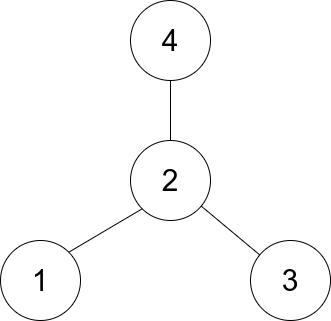##### Welcome to Subscribe On Youtube

Formatted question description: https://leetcode.ca/all/1791.html

# 1791. Find Center of Star Graph

Medium

## Description

There is an undirected star graph consisting of n nodes labeled from 1 to n. A star graph is a graph where there is one center node and exactly n - 1 edges that connect the center node with every other node.

You are given a 2D integer array edges where each edges[i] = [u_i, v_i] indicates that there is an edge between the nodes u_i and v_i. Return the center of the given star graph.

Example 1:Input: edges = [[1,2],[2,3],[4,2]]

Output: 2

Explanation: As shown in the figure above, node 2 is connected to every other node, so 2 is the center.

Example 2:

Input: edges = [[1,2],[5,1],[1,3],[1,4]]

Output: 1

Constraints:

• 3 <= n <= 10^5
• edges.length == n - 1
• edges[i].length == 2
• 1 <= u_i, v_i <= n
• u_i != v_i
• The given edges represent a valid star graph.

## Solution

The given star graph is actually a tree, with n nodes and n - 1 edges. The center of the star graph has n - 1 edges connected to it, and each of the remaining nodes has 1 edge connected to it. Simply calculate the number of edges connected to each node, and return the node that has more than 1 edge connected to it.

• class Solution {
public int findCenter(int[][] edges) {
int n = edges.length + 1;
int[] connections = new int[n + 1];
for (int[] edge : edges) {
int node1 = edge, node2 = edge;
connections[node1]++;
connections[node2]++;
if (connections[node1] > 1)
return node1;
else if (connections[node2] > 1)
return node2;
}
return 0;
}
}

############

class Solution {
public int findCenter(int[][] edges) {
int a = edges, b = edges;
int c = edges, d = edges;
return a == c || a == d ? a : b;
}
}

• // OJ: https://leetcode.com/problems/find-center-of-star-graph/
// Time: O(1)
// Space: O(1)
class Solution {
public:
int findCenter(vector<vector<int>>& E) {
return E == E || E == E ? E : E;
}
};

• class Solution:
def findCenter(self, edges: List[List[int]]) -> int:
return edges if edges in edges else edges

############

# 1791. Find Center of Star Graph
# https://leetcode.com/problems/find-center-of-star-graph/

class Solution:
def findCenter(self, edges: List[List[int]]) -> int:
n = len(edges)
mp = collections.defaultdict(int)

for a,b in edges:
mp[a] += 1
mp[b] += 1

for key in mp:
if mp[key] == n:
return key

return -1


• func findCenter(edges [][]int) int {
a, b := edges, edges
c, d := edges, edges
if a == c || a == d {
return a
}
return b
}

• function findCenter(edges: number[][]): number {
for (let num of edges) {
if (edges.includes(num)) {
return num;
}
}
}


• /**
* @param {number[][]} edges
* @return {number}
*/
var findCenter = function (edges) {
const [a, b] = edges;
const [c, d] = edges;
return a == c || a == d ? a : b;
};


• impl Solution {
pub fn find_center(edges: Vec<Vec<i32>>) -> i32 {
if edges == edges || edges == edges {
return edges;
}
edges
}
}